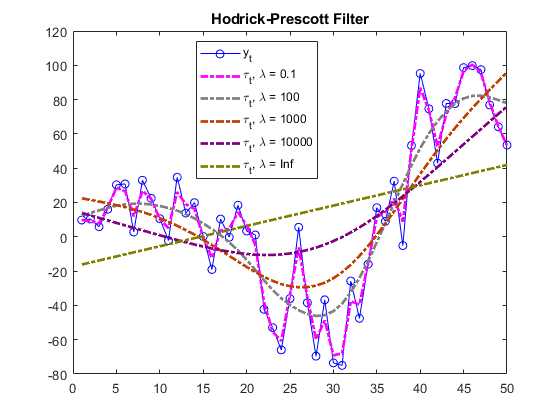hpfilter

Hodrick-Prescott filter for trend and cyclical components

Description

example

hpfilter(Y) plots the data of the time series variables (columns) of Y and their respective trend components computed by the Hodrick-Prescott Filter. The smoothing parameter is 1600, which is appropriate for quarterly periodicity. hpfilter plots all time series and their respective trend components on the same axes.

hpfilter(Y,smoothing) applies the Hodrick-Prescott filter smoothing parameter smoothing.

Trend = hpfilter(___) returns the trend components Trend of the time series variables using any of the input argument combinations in the previous syntaxes.

[Trend,Cyclical] = hpfilter(___) also returns the cyclical components Cyclical.

Examples

collapse all

Plot the cyclical component of the US post-WWII seasonally-adjusted real gross national poroduct (GNP). Specify smoothing of 1600, which is appropriate for quarterly data.

gnpDate = dates;
realgnp = DataTable.GNPR;
[~,c] = hpfilter(realgnp,1600);

plot(gnpDate,c)
axis tight
ylabel('Real GNP cyclical component')Input Arguments

collapse all

Time series data, specified as a numeric vector of length numObs or a numObs-by-numSeries numeric matrix.

• A vector represents numObs observations of a single series or variable.

• A matrix represents numObs observations of numSeries series. Y(j,k) is the observed value of series k at time j. Observations within the same row occur simultaneously.

The last element or row contains the latest observation.

If any element of Y is NaN or Inf, hpfilter issues an error.

Data Types: double

Trend component smoothing parameter, specified as a nonnegative numeric scalar or a nonnegative numeric vector of length numSeries. For a numeric scalar, hpfilter applies smoothing to all series in Y. For a numeric vector, hpfilter applies smoothing(k) to series k in the data (Y(:,k)).

If smoothing(k) is 0, hpfilter does not smooth the trend component of series k. In this case, the following are true:

• Trend(:,k) = Y(:,k).

• Cyclical(:,k) = zeros(numObs,1).

If smoothing(k) is Inf, hpfilter applies the maximum smoothing. In this case, the following are true:

• Trend(:,k) is the linear time trend computed by least squares.

• Cyclical(:,k) is the detrended series.

As the magnitude of the smoothing parameter increases, Trend approaches the linear time trend.

Appropriate values of the smoothing parameter depend on the periodicity of the data. Although a best practice is to experiment with smoothing values for your data, these smoothing values are recommended :

• 14400 for monthly data

• 1600 for quarterly data

• 100 for yearly data

Example: 100

Data Types: double

Output Arguments

collapse all

Trend component τt of each series in the data, returned as a numeric vector or matrix with the same dimensions as Y.

Cyclical component ct of each series in the data, returned as a numeric vector or matrix with the same dimensions as Y.

collapse all

Hodrick-Prescott Filter

The Hodrick-Prescott filter decomposes an observed time series yt (Y) into a trend component τt (Trend) and a cyclical component ct (Cyclical) such that yt = τt + ct.

The objective function of the filter is

$f\left({\tau }_{t}\right)=\sum _{t=1}^{T}{\left({y}_{t}-{\tau }_{t}\right)}^{2}+\lambda \sum _{t=2}^{T-1}{\left[\left({\tau }_{t+1}-{\tau }_{t}\right)-\left({\tau }_{t}-{\tau }_{t-1}\right)\right]}^{2},$

where:

• T is the sample size.

• λ is the smoothing parameter (smoothing).

• ytτt = ct.

The programming problem is to minimize the objective function over τ1,…,τT. The objective penalizes the sum of squares for the cyclical component with the sum of squares of second-order differences for the trend component (trend acceleration penalty). If λ = 0, the minimum of the objective is 0 with τt = yt for all t. As λ increases, the penalty for a flexible trend increases, resulting in an increasingly smoother trend. When λ is arbitrarily large, the trend acceleration approaches 0, resulting in a linear trend.

This figure shows the effects of increasing the smoothing parameter on the trend component for a simulated series.The filter is equivalent to a cubic spline smoother, where the smoothed component is τt.

Tips

• For high-frequency series, the Hodrick-Prescott filter can produce anomalous endpoint effects. In this case, do not extrapolate the series using the results of the filter.

 Hodrick, Robert J., and Edward C. Prescott. "Postwar U.S. Business Cycles: An Empirical Investigation." Journal of Money, Credit and Banking 29, no. 1 (February 1997): 1–16. https://doi.org/10.2307/2953682.